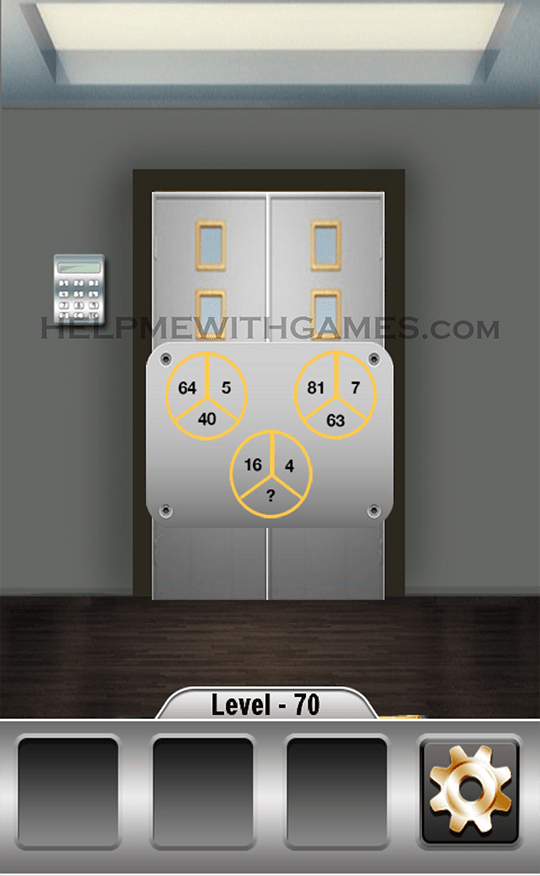# 100 Weird Doors – Walkthrough – Level 70100 Weird Doors Impossible & Unravel Escape Puzzle is developed by Fun Games Tree

All the answers here! The main goal in 100 Weird Doors is to escape the room.
Using all the possibilities of the device, you need to open each of the 100 doors using your brain and imagination.
Search for hidden objects and solve puzzles to make it through each doors!

Solution for the level 70:

You have 3 circles each divided by 3. You need to find the ?
If you take the upper left part of each circle and add them together you get.
64+81+16=161
If you take the upper right part of each circle and add them together you get.
5+7+4=16
The last part, the bottom part of each circle equals the difference of these two.
So, 161-16=145.
Take this number and remove the two numbers that you know from the bottom part of the circles.
145-40=105
105-63=42
The code is 42Click here to go back to the list of all the levels

This site uses Akismet to reduce spam. Learn how your comment data is processed.